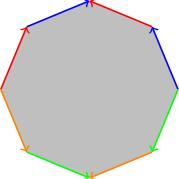# 5.02 Fundamental group of a CW complex

Below the video you will find accompanying notes and some pre-class questions.

# Notes

## Example of the 2-torus revisited

(0.00) In the previous video, we saw how to use Van Kampen's theorem to compute the fundamental group of the 2-torus. The result was $\langle a,b\ |\ b^{-1}a^{-1}ba=1\rangle.$ There is a CW structure on the 2-torus (square with opposite sides identified) with one 0-cell (vertex), two 1-cells (edges) and one 2-cell (the square itself). It is no coincidence that the presentation we obtained has:

• one generator for each 1-cell,
• one relation for each 2-cell: the relation comes from looking at the boundary of the 2-cell.

## Fundamental group of a CW complex

(1.24) If $$X$$ is a CW complex with one 0-cell (for simplicity) then $$\pi_1(X)$$ has a presentation where the generators are the 1-cells and the relations come from the 2-cells. More precisely,
• each 1-cell is a loop (as both ends attach to the unique 0-cell). These loops generate $$\pi_1(X)$$;
• (3.05) the boundary of each 2-cell $$e$$ gives a loop $$\partial e$$ in the 1-skeleton which we can write as a word in the 1-cells; since this loop is nullhomotopic in $$e$$ we need the relation $$\partial e=1$$ in $$\pi_1(X)$$. These relations suffice to give a presentation of $$\pi_1(X)$$.
(5.18) Note that this theorem is about cell complexes of /any/ dimension; in other words, the 3-cells, 4-cells, etc. do not affect the fundamental group.
(5.38) Let $$X^1$$ be the 1-skeleton of $$X$$. This is obtained by attaching 1-cells to a point, so $$X^1$$ is just a wedge of 1-cells $$X^1=\bigvee_{1\mbox{-cells}}S^1$$. By induction, using the computation from last time, $$\pi_1(X^1)=\bigstar_{1\mbox{-cells}}\mathbf{Z}$$ (i.e. the free product of copies of the integers, one for each 1-cell, in other words, the group with one generator for each 1-cell and no relations).

(7.42) What happens when we attach a 2-cell?

Claim: If $$Y$$ is any space and $$Z$$ is obtained from $$Y$$ by attaching a 2-cell $$e$$ with some attaching map $$\varphi$$ then $$\pi_1(Z)=\pi_1(Y)/N(\partial e)$$, where $$N(\partial e)$$ is the normal subgroup generated by the boundary of $$e$$ (that is $$[\varphi]\in\pi_1(Y)$$). This is equivalent to imposing the relation $$\partial e=1$$.

(9.48) Proof of claim: This follows from Van Kampen's theorem. If we decompose $$Z=U\cup V$$ where $$U$$ is the interior of the 2-cell $$int(e)$$ and $$V$$ is the union $$Y\cup (e\setminus\{0\})$$ (that is, $$V$$ is everything except one point in the interior of $$e$$).

• The intersection $$U\cap V$$ is $$e\setminus\{0\}$$, the punctured 2-cell. That is homotopy-equivalent to a circle.
• $$U$$ is contractible.
• $$V$$ is homotopy equivalent to $$Y$$ (retracting the punctured 2-cell down onto its boundary in $$Y$$).
(11.45) Van Kampen's theorem tells us that $$\pi_1(Z)=\pi_1(Y)\star_{\mathbf{Z}}\{1\}$$. In other words, we take $$\pi_1(Y)$$ and we add an amalgamated relation $$\partial e=1$$ ($$\partial e$$ generates $$\pi_1(U\cap V)$$: in $$\pi_1(U)$$ is becomes trivial and in $$\pi_1(V)$$ it becomes $$\partial e$$).

(13.28) What about adding higher dimensional cells? By the same argument, where $$e$$ is an $$n$$-cell with $$n>2$$, we get $\pi_1(Z)=\pi_1(Y)\star_{\{1\}}\{1\},$ because $$U\cap V$$ is now homotopy equivalent to the $$(n-1)$$-sphere, which is simply-connected (so there is no new amalgamated relation).

# Pre-class questions

1. Given that the quotient of the octagon by the identifications indicated in the figure below is a genus 2 surface, use Van Kampen's theorem to give a presentation for the fundamental group of a genus 2 surface.